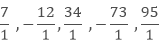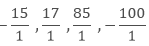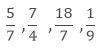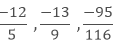×#### Thank you for registering.

One of our academic counsellors will contact you within 1 working day.

Click to Chat

1800-1023-196

+91-120-4616500

CART 0

• 0

MY CART (5)

Use Coupon: CART20 and get 20% off on all online Study Material

ITEM
DETAILS
MRP
DISCOUNT
FINAL PRICE
Total Price: Rs.

There are no items in this cart.
Continue Shopping• Complete JEE Main/Advanced Course and Test Series
• OFFERED PRICE: Rs. 15,900
• View Details

```Chapter 4: Rational Numbers Exercise – 4.1

Question: 1

Write down the numerator of each of the following rational numbers:

(i) -7/5

(ii)  15/(-4)

(iii)  (- 17)/(- 21)

(iv) 8/9

(v)  5

Solution:

Numerators are:

(i) -7

(ii)  15

(iii) -17

(iv) 8

(v) 5

Question: 2

Write down the denominator of each of the following rational numbers:

(i) - 4/5

(ii)  11/(-34)

(iii)  15/82

(iv) 15

(v)  0

Solution:

Denominators are:

(i)  5

(ii) - 34

(iii)  - 82

(iv) 1

(v)  1

Question: 3

Write down the rational number whose numerator is (-3) ×4, and whose denominator is (34 – 23) ×(7 – 4).

Solution:

According to the question:

Numerator = (-3) x 4 = - 12

Denominator = (34 – 23) x (7 – 4) =11 x 3 = 33

Therefore, Rational number = -12/32

Question: 4

Write the following rational numbers as integers:Solution:

Integers are 7, -12, 34, -73 and 95.

Question: 5

Write the following integers as rational numbers with denominator 1:

-15, 17, 85, -100

Solution:

Rational numbers of given integers with denominator 1 are:Question: 6

Write down the rational whose numerator is the smallest three digit number and denominator is the largest four digit number.

Solution:

Smallest three digit number = 100

Largest four digit number = 9999

Therefore rational number = 100/9999

Question: 7

Seperate positive and negative rational numbers from the following rational numbers:Solution:

Given rational numbers can be rewritten as:Thus, positive rational numbers are:Negative rational numbers are:Question: 8

Which of the following rational numbers are positive:

(i)  (- 8)/7

(ii)   9/8

(iii)  19/13

(iv) (- 21)/13

Solution:

The numbers can be rewritten as:

(i)  (- 8)/7

(ii)   9/8

(iii)  19/13

(iv) -21/13

Positive rational numbers are (ii) and (iii), i.e., 9/8 and (-19)/(-13)

Question: 9

Which of the following rational numbers are negative?

(i)  (- 3)/7

(ii) (- 5)/(- 8)

(iii) 9/(- 83)

(iv) 115/197

Solution:

The numbers can be rewritten as:

(i)  (- 3)/7

(ii)   (-5)/(- 8)

(iii)  9/(- 83)

(iv) 115/197

Negative rational numbers are (i) and (iii).
```### Course Features

• 728 Video Lectures
• Revision Notes
• Previous Year Papers
• Mind Map
• Study Planner
• NCERT Solutions
• Discussion Forum
• Test paper with Video Solution